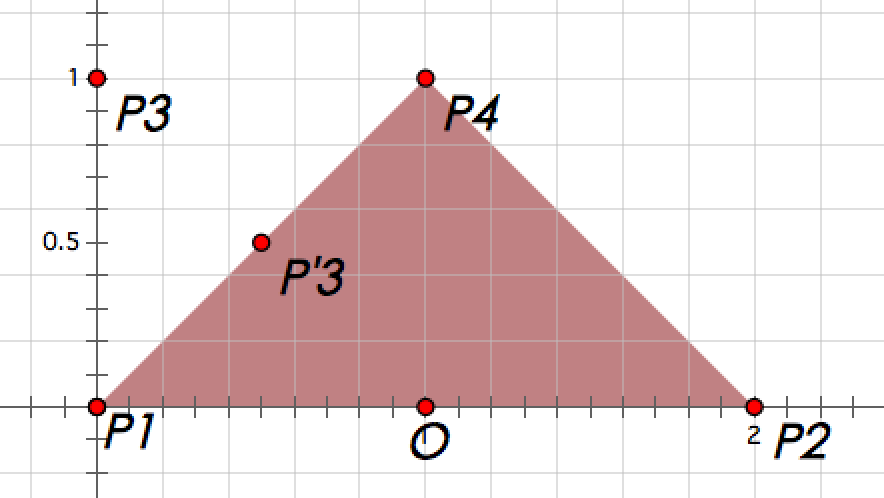# #413. 【ZJOI2018】保镖

• 换岗时，可怜和保镖们的位置可以被抽象成二维平面直角坐标系上的点。设可怜的位置为 $O$，保镖的位置为 $P_i$，换岗后的位置为 $P'_i$
• 在换岗前后，保镖和可怜的相对位置不变。即对于 $\forall i \in [1,n]$，$P'_i$ 在射线 $OP_i$ 上。
• 在换岗前后，保镖和可怜的距离变成了原来的倒数。即对于 $\forall i \in [1,n]$，$|OP_i||OP'_i|=1$。

• 对于一个简单多边形，它是凸多边形当且仅当它内部任意两点的连线在它的内部。
• 对于一个点集 $P$，它的凸包为包含所有点面积最小的没有三个连续顶点共线的凸多边形。

### 样例一

#### input

4
0 0 1 1
0 0
2 0
0 1
1 1



#### output

3.7853981633974474



#### explanation### 限制与约定

$1$$\leq3 2,3$$\leq4$
$4,5,6$$\leq50 7,8$$\leq350$
$9,10$$\leq2000$